1592698920

# Selection Sort Algorithm Visually Explained

The Selection Sort algorithm sorts an array by looking for the smallest item and moving it to the front of the list. That’s really all you have to know. Let’s jump into an example.
Selection Sort starts by setting the first value as the minimum value.
It then starts comparing it with each value in the array. If a lower value is found, the minimum value is updated, and the traversal continues until the end of the array is reached. During the first comparison, the value at index 1 is compared to the minimum value.

#programming #algorithms #computer-science #sorting-algorithms #selection-sort

## Buddha Community1592698920

## Selection Sort Algorithm Visually Explained

The Selection Sort algorithm sorts an array by looking for the smallest item and moving it to the front of the list. That’s really all you have to know. Let’s jump into an example.
Selection Sort starts by setting the first value as the minimum value.
It then starts comparing it with each value in the array. If a lower value is found, the minimum value is updated, and the traversal continues until the end of the array is reached. During the first comparison, the value at index 1 is compared to the minimum value.

#programming #algorithms #computer-science #sorting-algorithms #selection-sort1592620980

## Topological Sorting Visually Explained

Topological sorting is the result you get when you sort the tree in order of decreasing finishing times. What this means is that you run the Depth First Search algorithm and once it’s complete, you’ll have two sets of values: discovery times and finishing times. Finishing times sorted in reverse will generate the topologically sorted list.
DFS starts traversing the tree at vertex A. Since it has been discovered, we’ll mark it with the number 1. Number 1 is used to indicate that it has been discovered first.

#sorting-algorithms #algorithms #computer-science #topological-sort #programming1598525400

## Visualize an Interesting Sorting Algorithms With Python

There are various types of sorting algorithms out there and sometimes it becomes very difficult to understand their internal working without visualization. Hence I decided to visualize these sorting algorithms in python with the help of matplotlib.animations library.

NOTE:- In this article, we will also compute the number of operations performed and will be able to see the time complexity of the sorting algorithm.

As our purpose is to only visualize the sorting algorithms hence I will be using the `merge sort `for a demonstration but you should implement the rest of them in order to understand the differences among them.

Before we start coding, you must have `python 3.3 `or above installed because I have used the `yield from` feature or generator.

### Let’s Start:-

Firstly you need to import the given libraries. We have used the `random` module in order to generate a random array of numbers to be sorted. The `matplotlib pyplot` and `animation` modules will be used to animate the sorting algorithm.

``````import random
import matplotlib.pyplot as plt
import matplotlib.animation as anim
``````

Below the given `swap` function will be used to swap the elements in the given array. Defining a separate function is useful as it will be used exhaustively throughout different algorithms.

``````def swap(A, i, j):
a = A[j]
A[j] = A[i]
A[i] = a
## also in python A[i],A[j]=A[j],A[i]
``````

We have used `Merge Sort` to demonstrate this visualization because this is the most popular and one of the best sorting algorithms out there. Merge sort follows `Divide and Conquer` technique for sorting. It divides the array into two subarrays and each of these is sorted by calling the merge sort recursively on them. Our main focus is to visualize the algorithm hence I will not explain the working of it. The following code shows the merge sort. In order to get the intuition on how it works, one can follow this video on youtube jenny’s lecture.

``````def merge_sort(arr,lb,ub):
if(ub<=lb):
return
elif(lb<ub):
mid =(lb+ub)//2
yield from merge_sort(arr,lb,mid)
yield from merge_sort(arr,mid+1,ub)
yield from merge(arr,lb,mid,ub)
yield arr
def merge(arr,lb,mid,ub):
new = []
i = lb
j = mid+1
while(i<=mid and j<=ub):
if(arr[i]<arr[j]):
new.append(arr[i])
i+=1
else:
new.append(arr[j])
j+=1
if(i>mid):
while(j<=ub):
new.append(arr[j])
j+=1
else:
while(i<=mid):
new.append(arr[i])
i+=1
for i,val in enumerate(new):
arr[lb+i] = val
yield arr
``````

#python #programming #merge-sort #sorting-algorithms #visualization1593542940

## Heap Sort Explained

As you may know, heapsort is also one of the most asked sorting algorithms besides mergesort and quicksort and today we’re going to talk about it and I’ll try my best to explain it in much simpler terms as for a beginner it can be quite complicated more so if one has less knowledge about heaps. But fear not I’ve got your back. So now let’s get into it.

Before moving on to heap sort you need to know about the following terminologies to properly understand the working of heapsort.

1. Heaps
2. Heap Property
3. Heapify

So let’s begin by explaining the terms stated above

# 1. Heaps

Heap data structure is a**_ complete binary tree that follows the heapify property_**. So what’s a complete binary tee you might ask?. A complete binary tree follows these conditions.

• All nodes in every level of the tree are filled except the leaf nodes.
• The nodes are as far left as possible.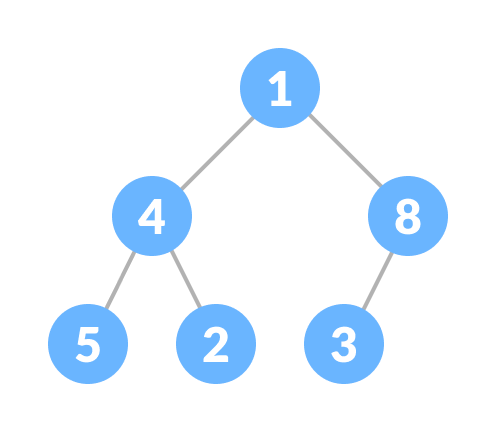Complete Binary Tree

The heap is shown below following the heap property and this is called Max heap. It has the same root and the child nodes but there is a slight difference. The Complete Binay Tree doesn’t care whether it’s root node is smaller or larger but it is of grave importance in case of the heap data structure.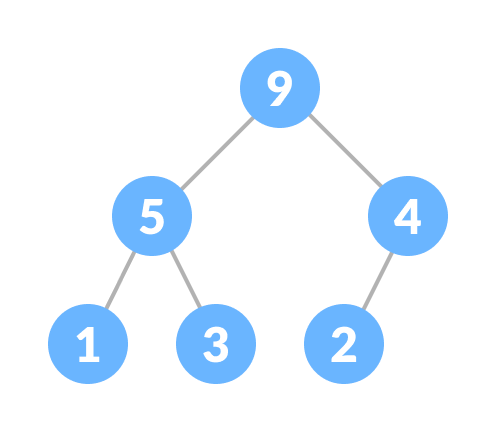Heap

# 2. Heap Property

While creating a heap it is essential to follow the heap property which states as follows:

• The heap where the parent node is always greater than the child nodes and provided that the root node is largest is called a Max-heap.Max Heap

• The heap in which the child node is always greater than it’s child nodes and the root node is the smallest node is called a Min-heap.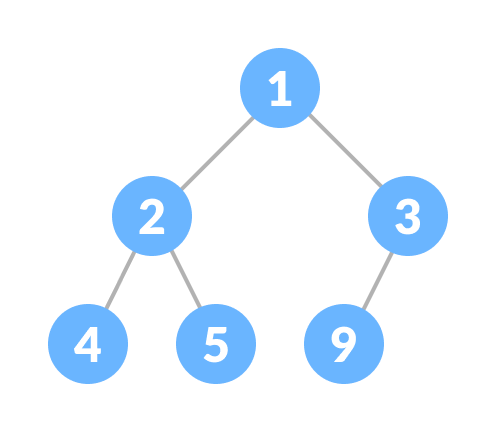Min heap

# 3. Heapify

Heapify is the** process of creating a heap data structure from a binary tree. **Now Heapify is the most important operation on a heap data structure as it helps to maintain the structure of the heap after several operations have been performed on the heap like(insertion, deletion). We will be using this operation a lot and it will come in handy while designing heap sort.

working of heapify is shown below

1. Select an input array.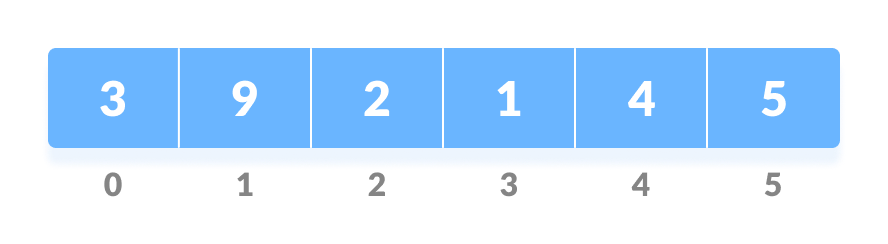Input array

2. Convert this array into a complete binary tree.

Complete binary tree created from the array

3. Create a heap from the binary tree.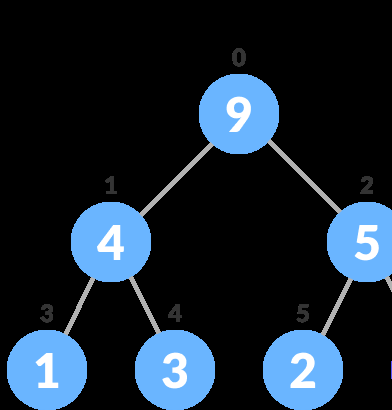After Heapify a max heap is created

Now that we’ve learned about all the important terms let’s start to create heap sort algorithm.

In a heap sort program, we’ll be given an unsorted array and we have to sort it. But heapsort works somewhat different than other sorting algorithms. By different, means the arrangement of each element after operations are performed. In this, the larger elements are sorted first in the last place then followed by the smaller elements, and at last, when there’s one element left that element would be the smallest and the last to go in it’s sorted place.

#sorting #sorting-algorithms #heap #heapsort #sort1592628360

## Heap Sort Algorithm Visually Explained

Now that we know how to create a max-heap, we’ll move into sorting an array of values using heap sort. To sort the array using heap sort, we’ll keep creating max-heaps. Each time a max-heap is created, the root is removed and is considered sorted. Let’s start with the following example.

#heapsort #computer-science #sorting-algorithms #programming #algorithms